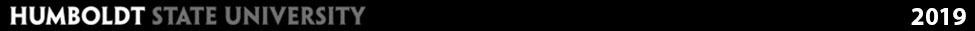# Scientific Notation & Metric Prefixes

## Scientific Notation

As mentioed in the previous section, when describing electromagnetic radiation we are often dealing with both very large and very small numbers. Therefore scientific notation is frequently used to write these numbers. Many of the variables for radiation equations are written in scientific notation, so you should become comfortable converting numbers to and from scientific notation as well as adding, multiplying and dividing numbers in scientific notation.

In scientific notation numbers are written in this format: a x 10b

The letter a stands for a decimal number, and the letter b stands for an exponent, or power of 10. For example, the number 5000 is written in scientific notation as 5 × 103. The number 0.03 is written as 3.0 × 10-2

### General Rules for Converting a Number into Proper Scientific Notation

1. Find all of the significant figures in the number. Re-write those digits as a number with 1 digit in front of the decimal point and the rest of the numbers after the decimal point (number greater than or equal to 1 but less than 10.)

2. Look at the new number you have written. Count the number of places you must move the decimal point in order to get back to where the decimal point was originally located.  This will be the numerical value of your exponent. If you have to move the decimal point to the right to get the original number, the exponent will be a positive number, if you have to move the decimal point to the left to get the original number, the exponent will be a negative number.

Example: Write 3040 in scientific notation.
The decimal should be between the 3 and 0, so move the decimal point 3 places to the left, so it becomes 3.04 x 103Example: Write 0.00012 in scientific notation
The decimal should be between the 1 and 2, so move the decimal point 4 places to the right, so it becomes 1.2 x 10-4### Converting from Scientific Notation to "Normal"

When converting from Scientific Notation to a standard or normal notation, use the value of the exponent to determine the number of places to move the decimal point. Move the decimal place to the right if the exponent is positive and move the decimal place to the left if the exponent is negative.

Example: Write 4.01 x 105 in"normal notation"
Since the exponent is positive we move the decimal point five places to the right, so it becomes 401,000For additional review on Scientific Notion see: Khan Academy: Scientific Notation Introduction and Review

### Working with Exponent & Numbers in Scientific Notation

Exponents and numbers in scientific notation are frequently used in remote sensing applications. Whether solving for the frequency of electromagnetic radiation, or using Wien's Law to determining the radiation of maximum radiation, you will find multiple variables with exponents. Therefore it's important that to understand the rules for working with exponents, this will also make solving the equations much easier.

#### Multiplication

To multiply two numbers expressed in scientific notation, simply multiply the coefficient (numbers in front) and then add the exponents.

Example: Calculate (5.1 x 104) x (2.5 x 103)
Multiply the coefficients, then add the exponents. In this case we end up with more that one digit in front of the decimal. Therefore we need to move the decimal to the left one place, which adds one to the exponent#### Division

To divide two numbers expressed in scientific notation, divide the coefficient (numbers out front) and subtract the exponents.

Example: Calculate (6.2 x 106) / (3.1 x 103)
Divide the coefficients, then subtract the exponents.There are also exponent rules that apply to powers and negative exponents. The table below is a quick reference guide to all of the commonly exponent rules and properties

### Table of Exponents rules and properties

Rule name Rule Example
Product rules a na m = a n+m 23 ⋅ 24 = 23+4 = 128
a nb n = (a b) n 32 ⋅ 42 = (3⋅4)2 = 144
Quotient rules a n / a m = a n-m 25 / 23 = 25-3 = 4
a n / b n = (a / b) n 43 / 23 = (4/2)3 = 8
Power rules (bn)m = bn⋅m (23)2 = 23⋅2 = 64
bnm = b(nm) 232 = 2(32)= 512
m√(bn) = b n/m 2√(26) = 26/2 = 8
b1/n = nb 81/3 = 38 = 2
Negative exponents b-n = 1 / bn 2-3 = 1/23 = 0.125

## Metric Prefixes and Conversions

In addition to the use of scientific notation, you will also see a variety of metric prefixes used to describe wavelength, frequency and energy.

### Table of Metric Prefixes

 Prefix Symbol Multiplier Exponential peta P 1,000,000,000,000,000 1015 tera T 1,000,000,000,000 1012 giga G 1,000,000,000 109 mega M 1,000,000 106 kilo k 1,000 103 hecto h 100 102 deca da 10 101 Base Unit 1 100 deci d 0.1 10-1 centi c 0.01 10-2 milli m 0.001 10-3 micro µ 0.000001 10-6 nano n 0.000000001 10-9 pico p 0.000000000001 10-12 femto f 0.000000000000001 10-15 atto a 0.000000000000000001 10-18

In order to perform the calculations for wavelgnth and frequency, we will need to convert frequency and wavelength units to base units of Hertz and Meters. Let's look at an example.

Example: Convert 610 nanometers to meters
First look at the table above to determine the conversion, in this case we see that 1 nanometer is equal to 10-9 meters. We simply multiply 610 by 10-9For more detailed examples, watch the below video.

← Back

Lab 2 →

Module Home